How to extract Time data from an Excel file column using Pandas?

• Last Updated : 02 Sep, 2020

Prerequisite: Regular Expressions in Python

In these articles, we will discuss how to extract Time data from an Excel file column using Pandas. Suppose our Excel file looks like below given image then we have to extract the Time from the Excel sheet column and store it into a new Dataframe column.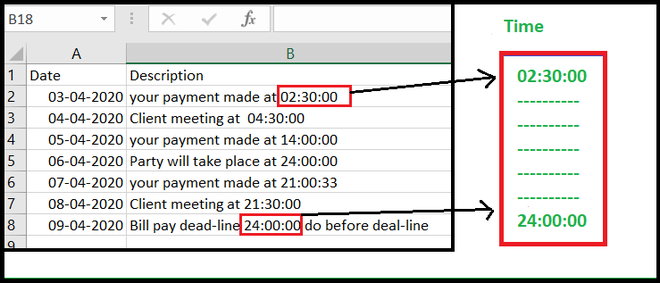Approach:

• Import the required module.
• Import data from Excel file.
• Make an extra column for store extracted time.
• Set Index for searching for extracting column.
• Define the pattern of Time format (HH: MM: SS).
• Search Time and assigning to the respective column in Dataframe.

Let’s see Step-By-Step-Implementation:

Step 1: Import the required module and read data from Excel file.

Python3

 # importing required moduleimport pandas as pd;import re;  # Read excel file and store in to DataFramedata = pd.read_excel("time_sample_data.xlsx");  print("Original DataFrame")data

Output: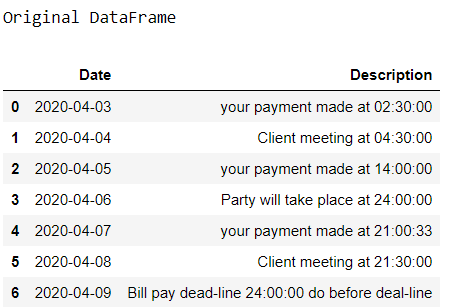Step 2: Make an extra column for storing Time data.

Python3

 # Create column for Timedata['New time'] = Nonedata

Output: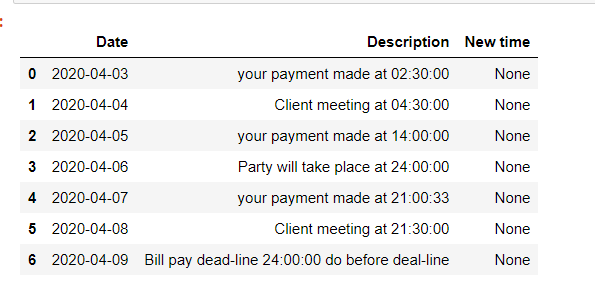Step 3: Set Index for searching

Python3

 # set indexindex_set = data.columns.get_loc('Description')index_time = data.columns.get_loc('New time')  print(index_set, index_time)

Output:

1 2

Step 4: Defining the Regular expression (regex) for the time.

Regex for time HH/ MM/ SS format:

[0-24]{2}\:[0-60]{2}\:[0-60]{2}.

Python3

 # define time patterntime_pattern = r'([0-24]{2}\:[0-60]{2}\:[0-60]{2})'

Step 5: Search Time and assigning to the respective column in Dataframe.

For searching the time using regex in a string we are using re.search() function of re library.

Python3

 # searching the entire DataFrame# with Time patternfor row in range(0, len(data)):        time = re.search(time_pattern,                     data.iat[row,index_set]).group()          data.iat[row, index_time] = time      print("Final DataFrame")    data

Output: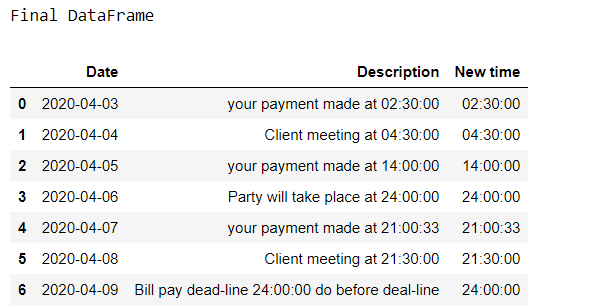Complete Code:

Python3

 # importing required moduleimport pandas as pd;import re;  data = pd.read_excel("time_sample_data.xlsx");print("Original DataFrame")print(data)  # Create column for Datedata['New time']= Noneprint(data)  # set indexindex_set= data.columns.get_loc('Description')index_time=data.columns.get_loc('New time')print(index_set,index_time)  # define the time pattern in HH:MM:SStime_pattern= r'([0-24]{2}\:[0-60]{2}\:[0-60]{2})'  #searching dataframe with time patternfor row in range(0, len(data)):    time= re.search(time_pattern,data.iat[row,index_set]).group()    data.iat[row,index_time] = time      print("\n Final DataFrame")    data

Output: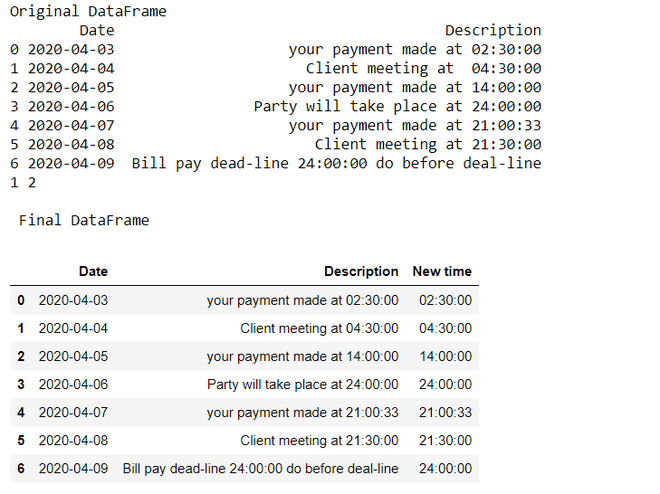Note: Before running this program, make sure you have already installed xlrd library in your Python environment.

My Personal Notes arrow_drop_up